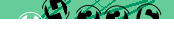# JavaScript: DHTML Quick Sort

This page demonstrates the Quick Sort algorithm. This is the most advanced algorithm of the four presented, but has some problems w.r.t. speed and the fact that it is not a 'stable' sort (see link at bottom of page for details). At this stage we've found the Shell Sort algorithm to be more efficient with web data.

idcolourrandom
1green861
2yellow86
3red687
4blue746
5orange916
6blue384
7blue433
8orange495
9green56
10orange159
11orange658
12blue855
13orange647
14red778
15green71
16blue247
17red502
18orange995
19red174
20green805
Sort by DESC?

## How does it work?

For a more detailed discussion on the sorting process, you can refer to the Bubble Sort page. The only difference between the two is the actual sorting algorithm, with the Quick Sort, in theory, being much faster:

``` // global variables var col = 0; var parent = null; var items = new Array(); var N = 0; function quicksort(m, n, desc) { if(n <= m+1) return; if((n - m) == 2) { if(compare(get(n-1), get(m), desc)) exchange(n-1, m); return; } i = m + 1; j = n - 1; if(compare(get(m), get(i), desc)) exchange(i, m); if(compare(get(j), get(m), desc)) exchange(m, j); if(compare(get(m), get(i), desc)) exchange(i, m); pivot = get(m); while(true) { j--; while(compare(pivot, get(j), desc)) j--; i++; while(compare(get(i), pivot, desc)) i++; if(j <= i) break; exchange(i, j); } exchange(m, j); if((j-m) < (n-j)) { quicksort(m, j, desc); quicksort(j+1, n, desc); } else { quicksort(j+1, n, desc); quicksort(m, j, desc); } } function sortTable(tableid, n, desc) { parent = document.getElementById(tableid); col = n; if(parent.nodeName != "TBODY") parent = parent.getElementsByTagName("TBODY"); if(parent.nodeName != "TBODY") return false; items = parent.getElementsByTagName("TR"); N = items.length; // quick sort quicksort(0, N, desc); }```

expand code box

This algorithm makes use of the get and compare function presented previously in the Bubble Sort demonstration, and the exchange function introduced in the Shell Sort demonstration.

The sorting functions presented so far have all been quite inefficient because they first have to read the DOM and then apply the various algorithms. To get around this limitation, each of the sorting algorithms has also been implemented using object-oriented (OOP) techniques.

All four methods (using OOP) are now available as external javascript files on the related page: DHTML Sorting Using OOP.

## References

<JavaScript

#### Send a message to The Art of Web: